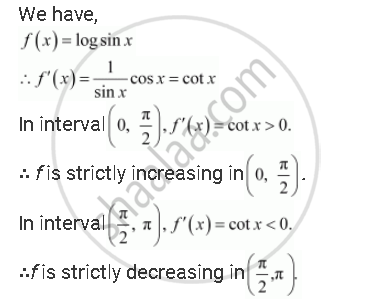Share

# Prove that the Function F Given By F(X) = Log Sin X Is Strictly Increasing on (0. Pi/2) And Strictly Decreasing on (Pi/2, Pi) - CBSE (Science) Class 12 - Mathematics

ConceptIncreasing and Decreasing Functions

#### Question

Prove that the function f given by f(x) = log sin x is strictly increasing on (0. pi/2) and strictly decreasing on (pi/2, pi)

#### SolutionIs there an error in this question or solution?

#### APPEARS IN

NCERT Solution for Mathematics Textbook for Class 12 (2018 to Current)
Chapter 6: Application of Derivatives
Q: 16 | Page no. 206

#### Video TutorialsVIEW ALL 

Solution Prove that the Function F Given By F(X) = Log Sin X Is Strictly Increasing on (0. Pi/2) And Strictly Decreasing on (Pi/2, Pi) Concept: Increasing and Decreasing Functions.
S